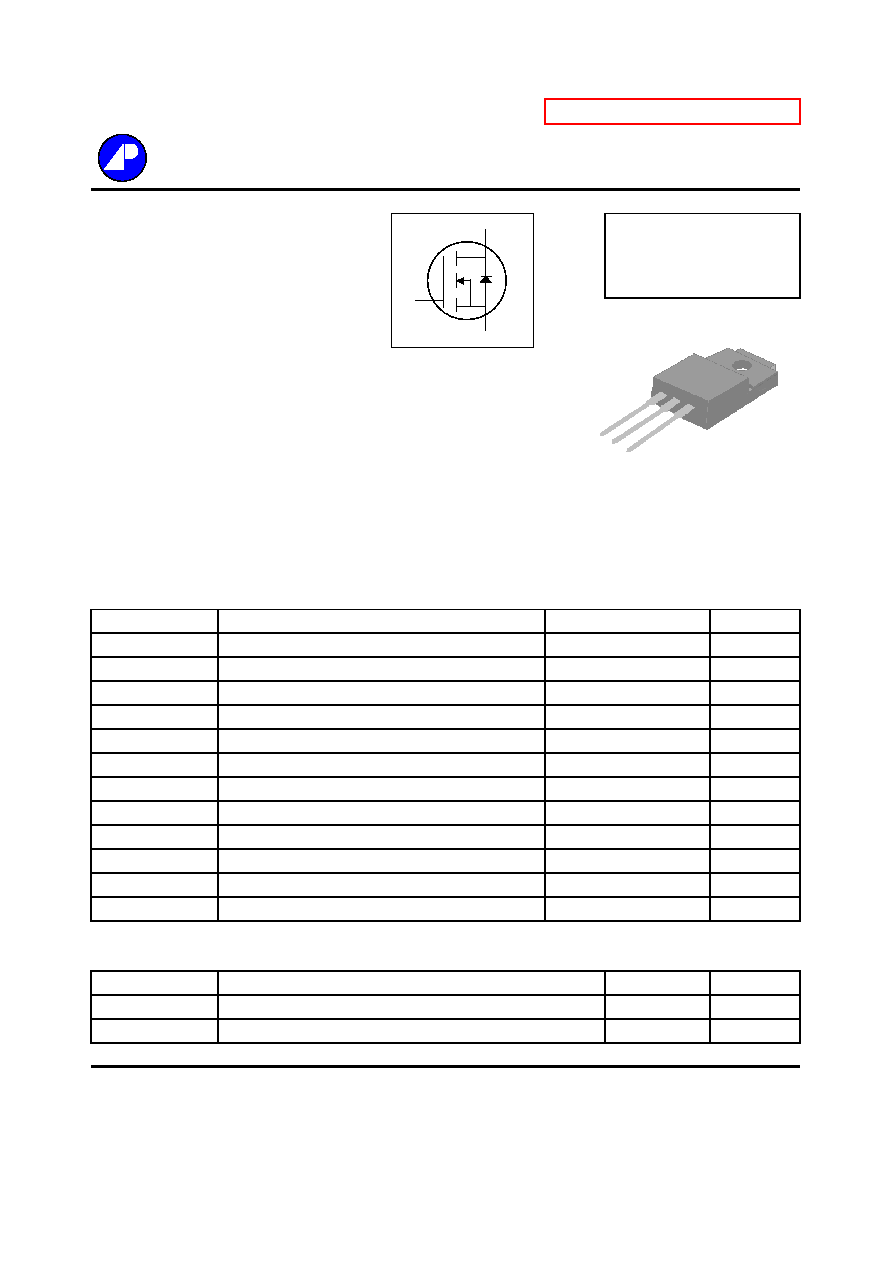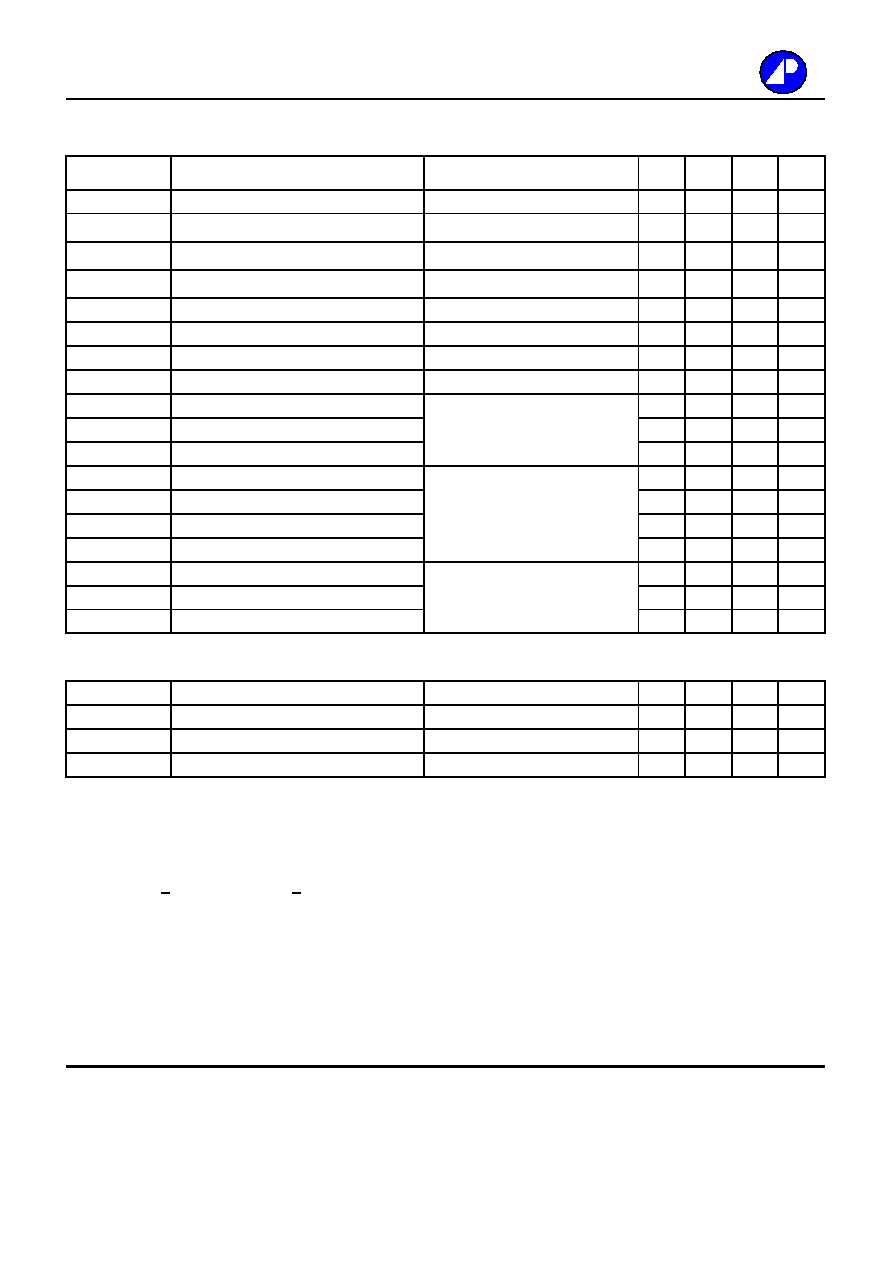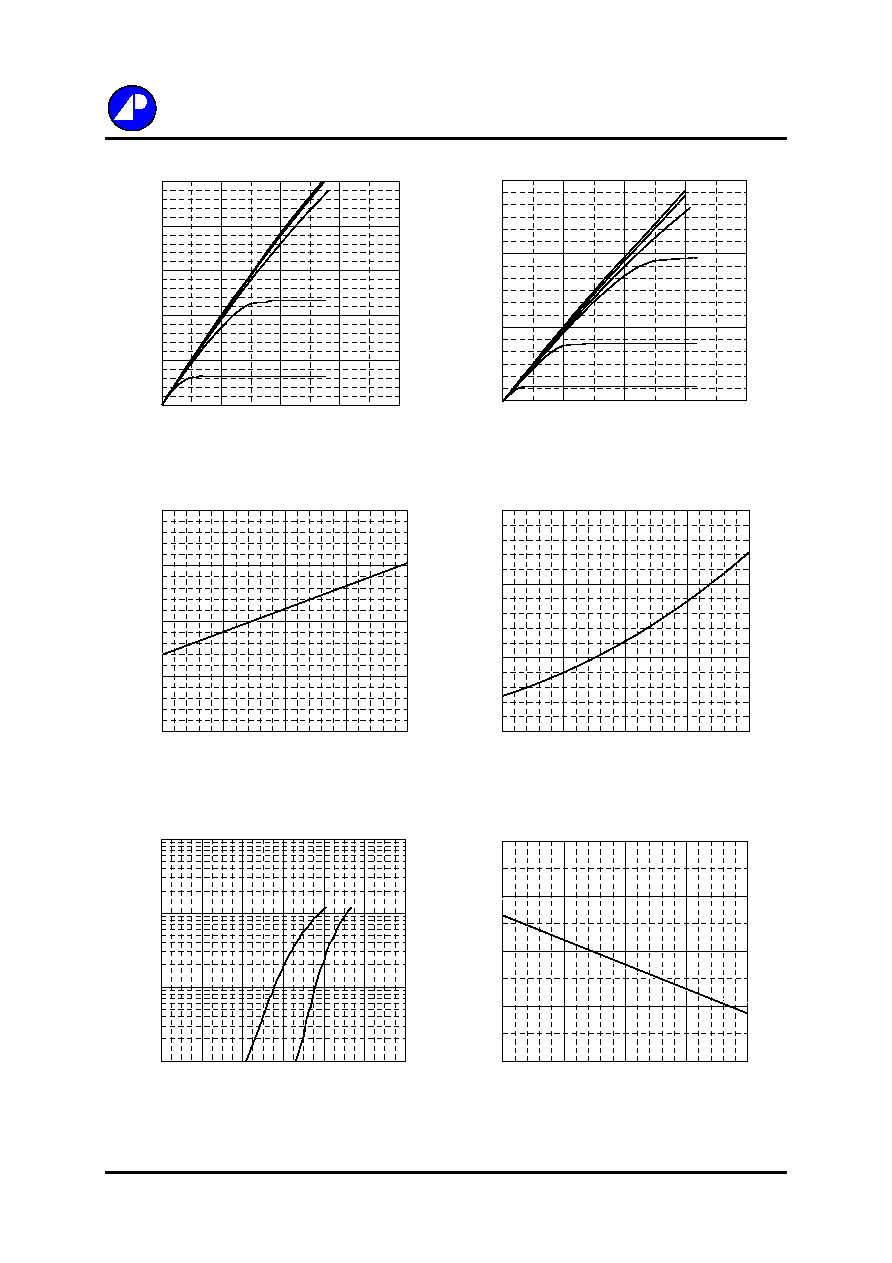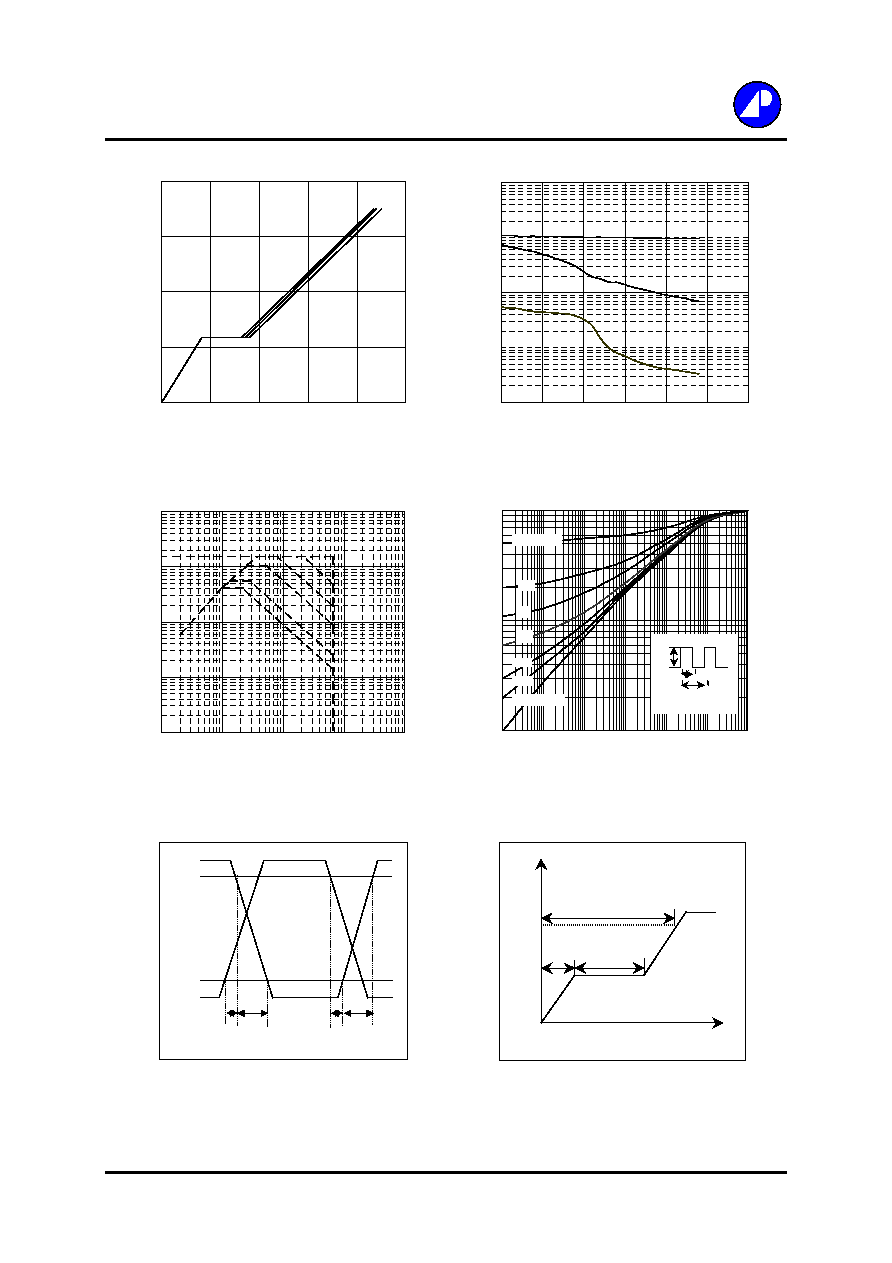Search datasheet (1.687.043 components) Search fieldPart namePart descriptionAbout site Manufacturers list ChipFindIC search engine AllXrefCross-reference database### Datasheet: AP04N70BF-A (Advanced Power Electronics Corp.)

N-channel Enhancement Mode Power Mosfet
Download:PDFZIPN-CHANNEL ENHANCEMENT MODE
Electronics Corp.
POWER MOSFET
Dynamic dv/dt Rating
BV
DSS
650V
Repetitive Avalanche Rated
R
DS(ON)
2.4
Fast Switching
I
D
4A
Simple Drive Requirement
RoHS Compliant
Description
Absolute Maximum Ratings
Symbol
Units
V
DS
Drain-Source Voltage
V
V
GS
Gate-Source Voltage
V
I
D
@T
C
=25
Continuous Drain Current, V
GS
@ 10V
A
I
D
@T
C
=100
Continuous Drain Current, V
GS
@ 10V
A
I
DM
Pulsed Drain Current
1
A
P
D
@T
C
=25
Total Power Dissipation
W
W/
E
AS
Single Pulse Avalanche Energy
2
mJ
I
AR
Avalanche Current
A
E
AR
Repetitive Avalanche Energy
mJ
T
STG
T
J
Operating Junction Temperature Range
Thermal Data
Symbol
Value
Units
Rthj-c
Thermal Resistance Junction-case
Max.
3.8
/W
Rthj-a
Thermal Resistance Junction-ambient
Max.
65
/W
Data & specifications subject to change without notice
4
4
15
33
Linear Derating Factor
0.26
-55 to 150
Parameter
AP04N70BF-A
100
Rating
650
Pb Free Plating Product
Parameter
200704051-1/4
�30
4
2.5
Storage Temperature Range
-55 to 150
G
D
S
G
D
S
TO-220FM
AP04N70 series are specially designed as main switching devices for
universal 90~265VAC off-line AC/DC converter applications. TO-220FM
type provide high blocking voltage to overcome voltage surge and sag
in the toughest power system with the best combination of fast
switching,ruggedized design and cost-effectiveness.
The TO-220FM package is universally preferred for all commercial-industrial
applications. The device is suited for switch mode power supplies ,DC-AC
converters and high current high speed switching circuits.Electrical Characteristics@T
j
=25
o
C(unless otherwise specified)
Symbol
Parameter
Test Conditions
Min.
Typ. Max. Units
BV
DSS
Drain-Source Breakdown Voltage
V
GS
=0V, I
D
=1mA
650
-
-
V
B
V
DSS
/T
j
Breakdown Voltage Temperature Coefficient
Reference to 25
, I
D
=1mA
-
0.6
-
V/
R
DS(ON)
Static Drain-Source On-Resistance
V
GS
=10V, I
D
=2A
-
-
2.4
V
GS(th)
Gate Threshold Voltage
V
DS
=V
GS
, I
D
=250uA
2
-
4
V
g
fs
Forward Transconductance
V
DS
=10V, I
D
=2A
-
2.5
-
S
I
DSS
Drain-Source Leakage Current (T
j
=25
o
C)
V
DS
=600V, V
GS
=0V
-
-
10
uA
Drain-Source Leakage Current (T
j
=150
o
C)
V
DS
=480V
,
V
GS
=0V
-
-
100
uA
I
GSS
Gate-Source Leakage
V
GS
=�30V
-
-
�100
nA
Q
g
Total Gate Charge
3
I
D
=4A
-
16.7
-
nC
Q
gs
Gate-Source Charge
V
DS
=480V
-
4.1
-
nC
Q
gd
Gate-Drain ("Miller") Charge
V
GS
=10V
-
4.9
-
nC
t
d(on)
Turn-on Delay Time
3
V
DD
=300V
-
11
-
ns
t
r
Rise Time
I
D
=4A
-
8.3
-
ns
t
d(off)
Turn-off Delay Time
R
G
=10
,
V
GS
=10V
-
23.8
-
ns
t
f
Fall Time
R
D
=75
-
8.2
-
ns
C
iss
Input Capacitance
V
GS
=0V
-
950
-
pF
C
oss
Output Capacitance
V
DS
=25V
-
65
-
pF
C
Reverse Transfer Capacitance
f=1.0MHz
-
6
-
pF
Source-Drain Diode
Symbol
Parameter
Test Conditions
Min.
Typ. Max. Units
I
S
Continuous Source Current ( Body Diode )
V
D
=V
G
=0V , V
S
=1.5V
-
-
4
A
I
SM
Pulsed Source Current ( Body Diode )
1
-
-
15
A
V
SD
Forward On Voltage
3
T
j
=25
, I
S
=4A, V
GS
=0V
-
-
1.5
V
Notes:
1.Pulse width limited by safe operating area.
2.Starting Tj=25
o
C , V
DD
=50V , L=25mH , R
G
=25
, I
AS
=4A.
3.Pulse width <300us , duty cycle <2%.
AP04N70BF-A
2/4Fig 1. Typical Output Characteristics
Fig 2. Typical Output Characteristics
Fig 3. Normalized BV
DSS
v.s. Junction
Fig 4. Normalized On-Resistance
Temperature
v.s. Junction Temperature
Fig 5. Forward Characteristic of
Fig 6. Gate Threshold Voltage v.s.
Reverse Diode
Junction Temperature
3/4
AP04N70BF-A
0.8
0.9
1
1.1
1.2
-50
0
50
100
150
T
j
, Junction Temperature (
o
C)
N
o
rmalize
d
BV
DSS
(V
)
0
1
2
3
-50
0
50
100
150
T
j
, Junction Temperature (
o
C )
N
o
rmalize
d
R
DS(ON)
I
D
=2A
V
G
=10V
0
0.6
1.2
1.8
0
3
6
9
12
V
DS
, Drain-to-Source Voltage (V)
I
D
, Dra
i
n C
u
rre
nt
(
A
)
T
C
=150
o
C
V
G
=3.5V
10V
6.0V
5.0V
4.5V
4.0V
0
0.5
1
1.5
2
2.5
0
2
4
6
8
V
DS
, Drain-to-Source Voltage (V)
I
D
, Dr
a
i
n C
u
r
r
e
nt
(A
)
T
C
=25
o
C
V
G
=4.0V
10V
6.0V
5.0V
4.5V
0.1
1
10
100
0
0.2
0.4
0.6
0.8
1
1.2
V
SD
(V)
I
S
(A
)
T
j
= 25
o
C
T
j
=150
o
C
1
2
3
4
5
-50
0
50
100
150
T
j
, Junction Temperature (
o
C )
V
GS(t
h)
(V
)Fig 7. Gate Charge Characteristics
Fig 8. Typical Capacitance Characteristics
Fig 9. Maximum Safe Operating Area
Fig 10. Effective Transient Thermal Impedance
Fig 11. Switching Time Waveform
Fig 12. Gate Charge Waveform
4/4
AP04N70BF-A
0.01
0.1
1
10
100
1
10
100
1000
10000
V
DS
(V)
I
D
(A
)
T
c
=25
o
C
Single Pulse
10us
100us
1ms
10ms
100ms
0.01
0.1
1
0.00001
0.0001
0.001
0.01
0.1
1
10
t , Pulse Width (s)
N
o
rmalize
d
T
h
e
r
mal Re
spon
se
(
R
th
jc
)
P
DM
Duty factor = t/T
Peak T
j
= P
DM
x R
thjc
+ T
C
t
T
0.02
0.01
0.05
0.1
0.2
Duty factor=0.5
Single Pulse
0
4
8
12
16
0
5
10
15
20
25
Q
G
, Total Gate Charge (nC)
V
GS
,
G
a
te
to S
o
u
r
c
e
Voltage
(
V
)
I
D
=4A
V
DS
=320V
V
DS
=400V
V
DS
=480V
1
100
10000
1
6
11
16
21
26
31
V
DS
, Drain-to-Source Voltage (V)
C (
p
F)
f=1.0MHz
C
iss
C
oss
C
t
d(on)
t
r
t
d(off)
t
f
V
DS
V
GS
10%
90%
Q
V
G
10V
Q
GS
Q
GD
Q
G
Charge© 2020 • ICSheet• Contact form• Main page